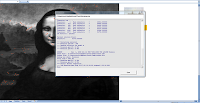## Wednesday, December 17, 2008

### Mona Lisa assignment in GAMS

This is the assignment problem described in Donald E. Knuth, "The Stanford GraphBase: A Platform for Combinatorial Computing", ACM Press, 1993. The gray scale data was generated by lisa(360,250,255,0,360,0,250,0,22950000) and is used to color (small) Excel cells.

The GAMS model is as follows:

``\$ontext     Assignment problem for lisa(360,250,255,0,360,0,250,0,22950000)     Erwin Kalvelagen, March 2002     Reference: Donald E. Knuth, "The Stanford GraphBase:        A Platform for Combinatorial Computing", ACM Press, 1993.    \$offtext    set i 'rows' /0*359/;set j 'columns' /0*249/;  \$if exist input.gdx \$call 'rm -f input.gdx'\$if exist output.xls \$call 'rm -f output.xls'  \$call '=gdxxrw.exe i=input.xls par=c rng=Data!A1 rdim=1 cdim=1' parameter c(i,j);\$gdxin input.gdx\$load c  equations    objective 'maximize highlighted parts'    column(j) 'column sum'    row(i)    'row sum'; variables    z         'objective variable'    x(i,j)    'pixels'; binary variable x; objective..      z =e= sum((i,j),c(i,j)*x(i,j));row(i)..         sum(j, x(i,j)) =l= 1;column(j)..      sum(i, x(i,j)) =e= 1; model m /all/;solve m using rmip maximizing z; set solution(i,j);solution(i,j)\$(x.l(i,j)>0.5) = yes;execute_unload 'output.gdx',solution; execute '=gdxxrw.exe i=output.gdx o=output.xls set=solution rng=Solution!A1 rdim=2 cdim=0'``

This model is stored inside the Excel spreadsheet. The Excel front-end will export the model and the input data to a temp directory. Then GAMS is called, and finally the resulting solution data is read. To make it possible to use gdxxrw inside the GAMS model, we use the following algorithm:

1. Write GAMS model to %TEMP%\MonaLisa.gms
2. Write Data sheet to %TEMP%\input.xls
3. Call gams to execute %TEMP%\MonaLisa.gms
4. Copy %TEMP%\output.xls to Solution sheet
5. Draw points in Solution sheet with different color
Note that GDXXRW cannot read/write directly to the spreadsheet that has VBA code running, neither can we make it shareable. This algorithm bypasses this problem by copying input and output data to external workbooks.

The model is a large but easy RMIP: 600 equations and 90,000 variables. The free LP solver CBC can solve this quickly: 0.6 seconds. Note: the model is too large to solve with MS Solver Foundation Express and Standard Editions (see: note). Using the Check button in the MS Solver Foundation Excel plug-in seems to indicate that the Excel binding is somewhat slow. In addition the plug-in does not provide a progress window (see second picture below) which is very useful for long running MIP models. Although the GAMS/EXCEL/VBA route requires more programming, for some classes of models this approach seems more flexible than the MS Solver Foundation Excel plug-in solution. Of course for ultimate flexibility at the cost of even more programming effort you can use the MS Solver Foundation .NET API's.